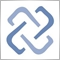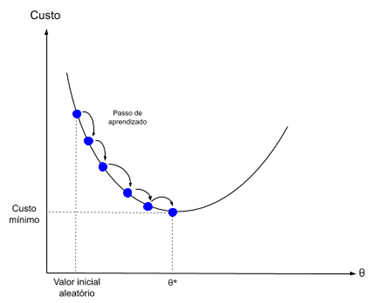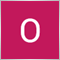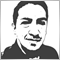# Discussion of article "Multilayer perceptron and backpropagation algorithm"Moderator
232664

New article Multilayer perceptron and backpropagation algorithm has been published:

The popularity of these two methods grows, so a lot of libraries have been developed in Matlab, R, Python, C++ and others, which receive a training set as input and automatically create an appropriate network for the problem. Let us try to understand how the basic neural network type works (including single-neuron perceptron and multilayer perceptron). We will consider an exciting algorithm which is responsible for network training - gradient descent and backpropagation. Existing complex models are often based on such simple network models.

Gradient descent is the process of minimizing a function in the direction of the gradients of the cost function.

This implies knowing the cost form, as well as the derivative, so that we can know the gradient from a certain point and can move in this direction, for example, downwards, towards the minimum value.In machine learning, we can use a technique that evaluates and updates the weights for each iteration, called Stochastic Gradient Descent. Its purpose is to minimize a model error in our training data.

The idea of this algorithm is that each training instance is shown to the model one at a time. The model creates a forecast for the training instance. Then an error is calculated and the model is updated to reduce the error in the next forecast.

This procedure can be used to find a set of model weights which produce the lowest error.

Author: Jonathan Pereira9

Awesome716

Superb... long ago I developed a pattern recognition algorithm based on statistical maths in some crude way... this ideas have revitalized and it is now much more accurate than ever. Thank you so much!